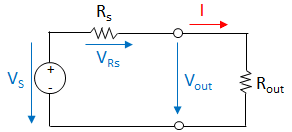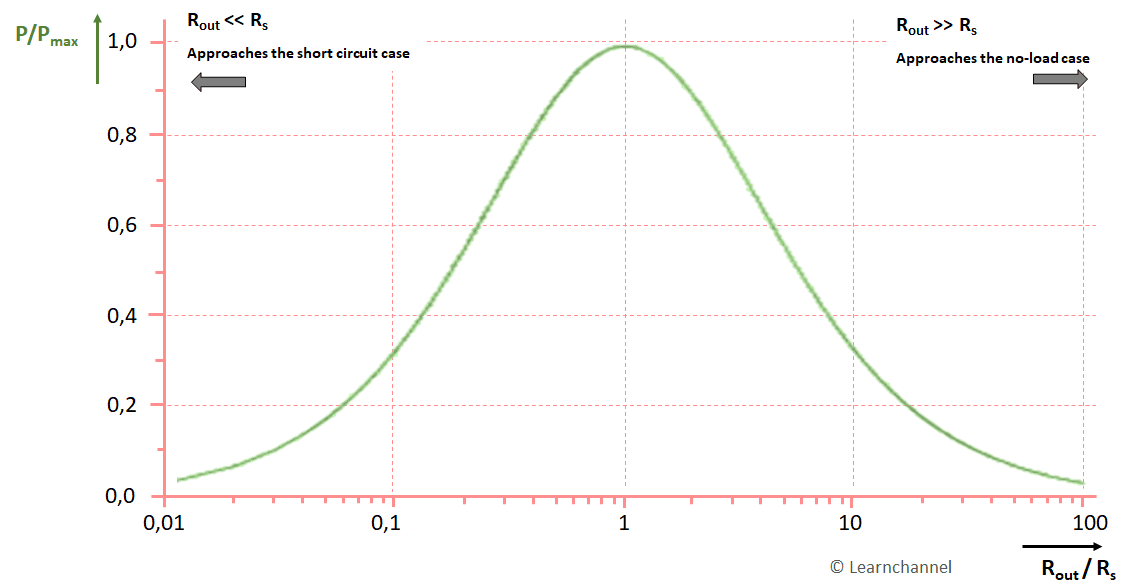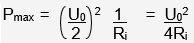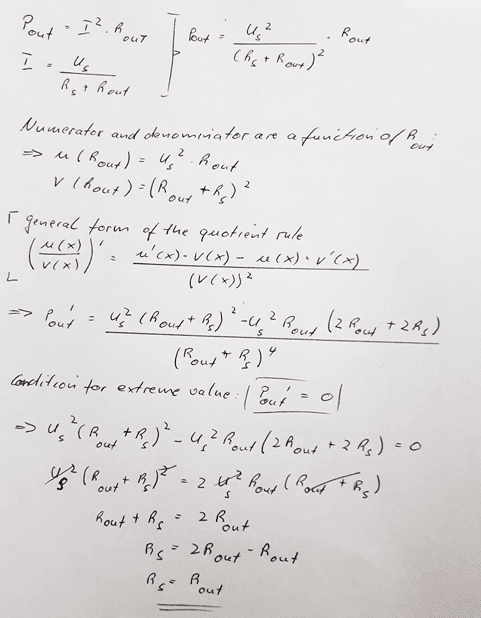# Power of a real voltage source, power adjustment

## Maximum Power from a Real Voltage Source, power adjustmentPractical Voltage Source

The power output from a real voltage source is calculated to:

Pout = Uout * I

In no-load operation, this power is zero, since no current flows through the load. In the case of a short circuit, no power is output either, since a high current flows, but the terminal voltage is zero. In this case, the current is limited solely by the internal resistance.Output power of a real voltage source as a function of the resistance ratio of external resistance Rout to source resistance Rs

The maximum power is emitted by the source when the external resistance Rout is equal to the internal resistance Rs of the voltage source: Rout = Rs. This case is called power adjustment. The maximum power is thus:The power dissipation of the internal resistance Ri is almost completely converted into thermal power (Pi=I2⋅Ri). It is therefore also responsible for the fact that, for example, batteries heat up during discharging or, in the case of accumulators, also during charging. In the event of a short circuit, this thermal power can lead to a fire.